# Visualizing Area of a Rectangle

Visual Number Talk to emerge and build an understanding of the relationship between the area of a rectangle and the array model.

## In This Set of Visual Number Talk Prompts…

Students will continue exploring the relationship between the area of rectangles and the array model. The array replaces the need to count individual units and makes it possible to calculate an area.

## String of Related Problems

Present the following rectangles one at a time. Students are asked to calculate the area of each rectangle in square units. Represent the student thinking symbolically. For example, if a student uses repeated addition, write the addition sentence on the board. If a student uses multiplication, write the multiplication sentence.

## Visual Math Talk Prompt #1

Students will be prompted with:

What is the area of the rectangle?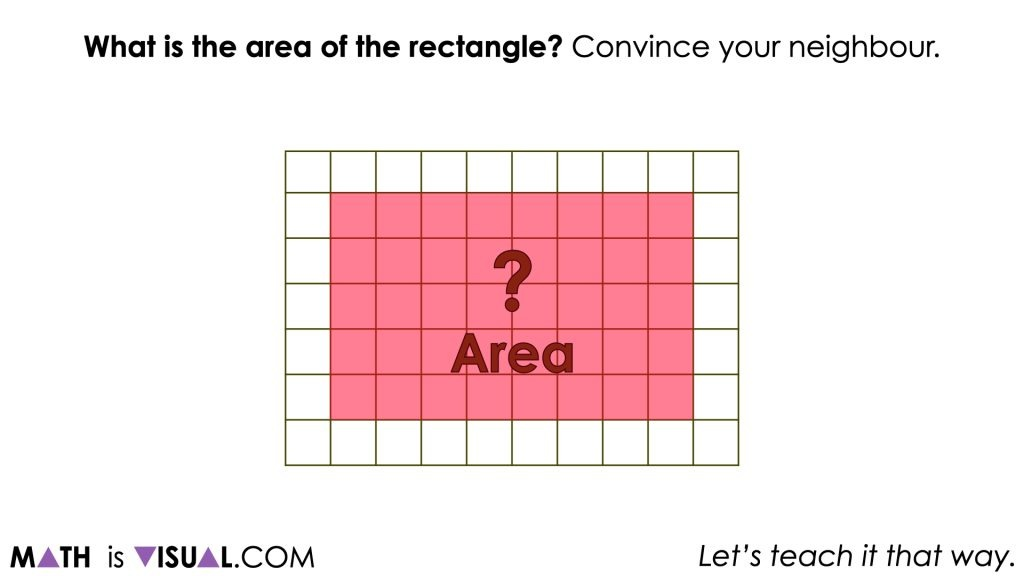The goal with the first visual number talk prompt in this string of problems is to ensure that students are understanding that determining a quantity of area involves counting 2-dimensional units. In this case, they are squares.

Although some students may choose to count each square 1-to-1, some students may recognize the pattern of rows and columns (an array) and use them to help make counting easier by skip counting.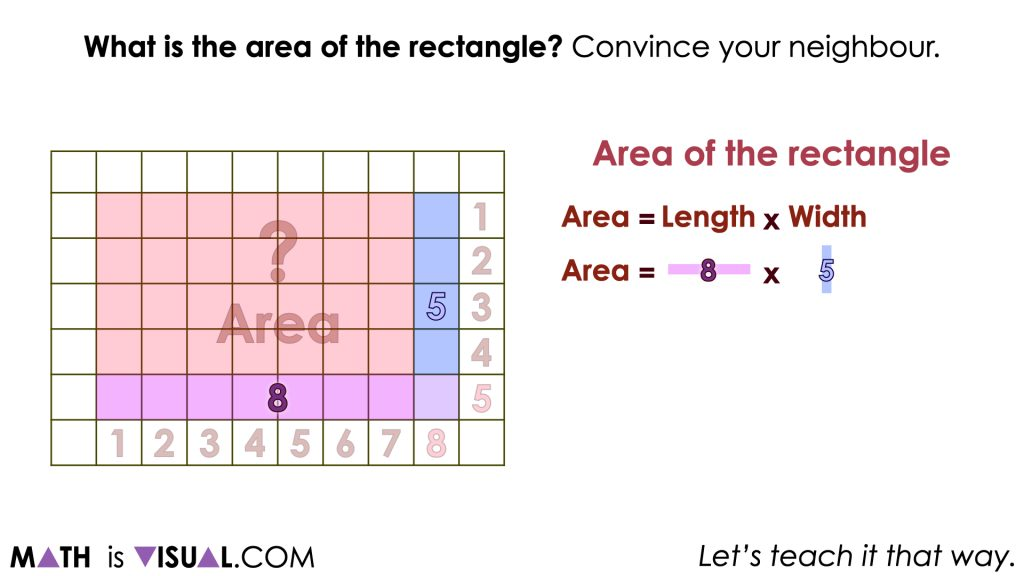At this point, we want to ensure we highlight student strategies such as one-to-one counting and skip counting and explicitly connect the skip counting strategies by rows and/or columns as a multiplication sentence.

For students that counted by rows, we can see that there were 5 rows of 8 units per row.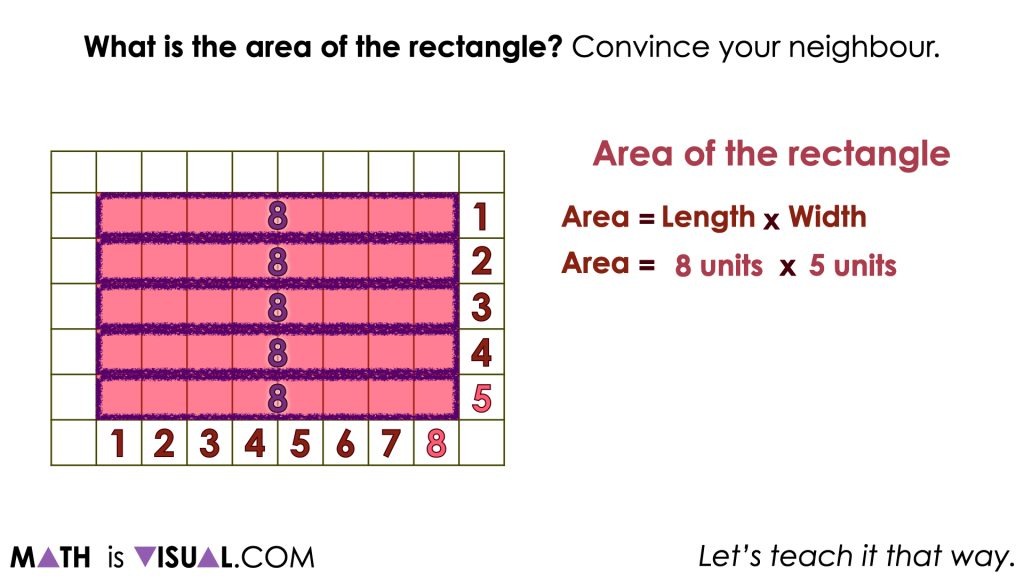For students counting by columns, we can highlight that there are 8 columns of 5 units per column.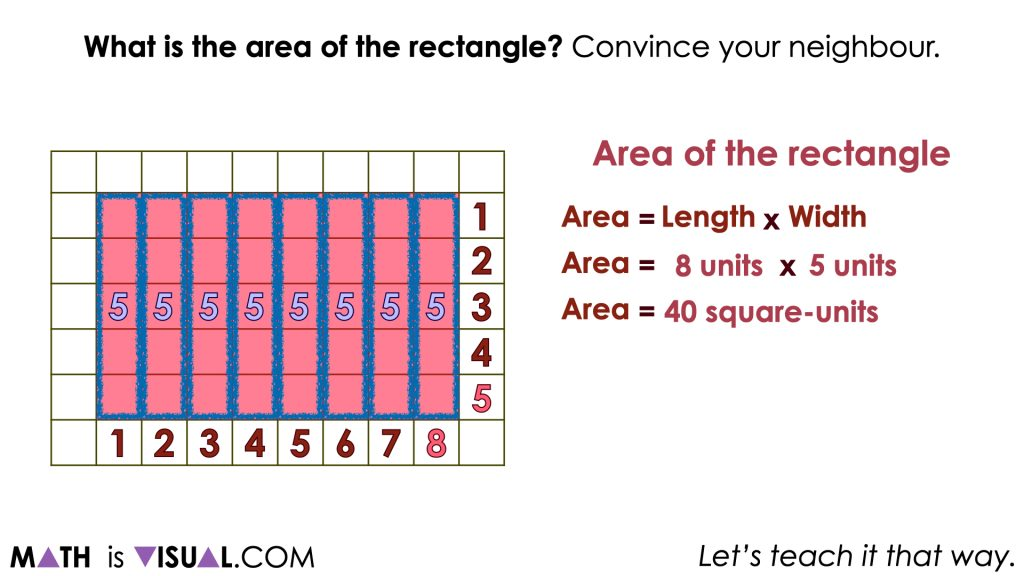Regardless of the strategy or strategies used by students, we hope all students are able to articulate that the area of this rectangle is 40 square units or 40 units-squared.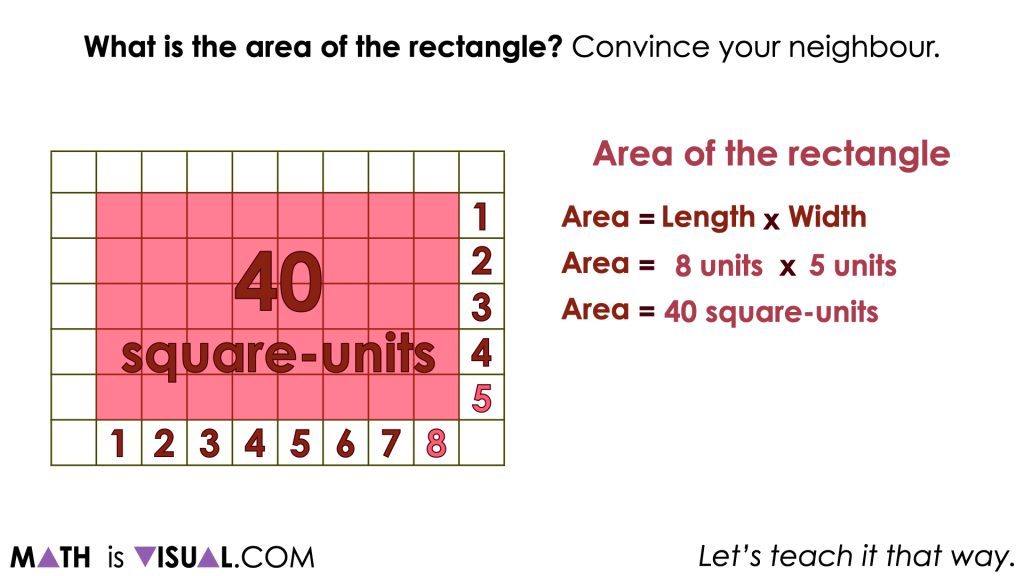## Want to Explore These Concepts & Skills Further?

Three (3) additional number talk prompts are available in Day 2 of the Grass is Greener problem based math unit that you can dive into now.

Why not start from the beginning of this contextual 5-day unit of real world lessons from the Make Math Moments Problem Based Units page.

Did you use this in your classroom or at home? How’d it go? Post in the comments!

Math IS Visual. Let’s teach it that way.